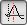## ShortIDDeclaration: TSpdc.ShortID (IncludeCaption: boolean): string; The function ShortID returns a short identifier for the spectral descriptor. This identifier consists of a two- or three-letter code followed by the most important parameters in square brackets. If the parameter IncludeCaption is TRUE the user-defined caption of the descriptor is appended to the identifier. Following are a few examples of such identifiers: ```TC [1369,1388...1350] ABL [3137...2974] VD [DC033+DC54] GCI [1579,1721...1480] PBL  GC [1824...1637,2] ```
The code conforms to the description of the spectral descriptors:

 Descriptor/Button Explanation ABLArea: sum of intensities between two reference points with the baseline subtracted. ALVArea: sum of all intensities between two reference points; the baseline is defined by the level of the reference point b1. ARWArea: the sum of intensities between two reference points; no baseline is subtracted. BBLThe logarithm of the ratio of two peak areas (with the baseline subtracted) weighted by the area of the second peak. BRWThe logarithm of the ratio of two peak areas weighted by the area of the second peak; no baseline is subtracted. CENCentroid: the position of the centroid of the spectral line within the boundaries b1 and b2. COOCorrelation with a positive triangle template peak within the boundaries b1 and b2. GCSignificant positive correlation (level of significance = 0.01) with a general Gaussian template peak. GCISignificant positive correlation (level of significance = 0.01) with an inverse general Gaussian template peak. PBLPeak: single intensity at a particular wavelength or mass; the baseline is subtracted. PLVPeak: single intensity at a particular wavelength or mass; the baseline is defined by the level of a single reference point b1. PRWRaw peak: single unprocessed intensity at a particular wavelength or mass; the baseline is not subtracted. RBLPeak ratio: the logarithm of the ratio of two peak intensities at two different wavelengths weighted by the intensity of the second peak. RLVPeak ratio: the logarithm of the ratio of two peak intensities at two different wavelengths weighted by the intensity of the second peak. RRWRaw peak ratio: the logarithm of the ratio of two peak intensities at two different wavelengths weighted by the intensity of the second peak. TCSignificant positive correlation (level of significance = 0.01) with a positive triangle template peak, multiplied by the signal area. TCISignificant positive correlation (level of significance = 0.01) with an negative triangle template peak, multiplied by the signal area. VARVariance of the first derivative of the spectral line within the boundaries b1 and b2.